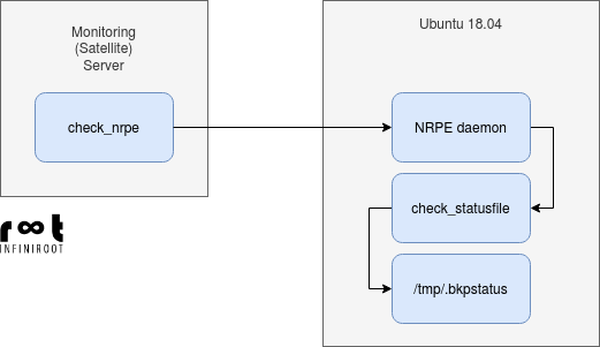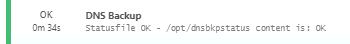# We sometimes write.

Of course we cannot always share details about our work with customers, but nevertheless it is nice to show our achievements and share some solutions.

# No such file or directory in /tmp although file exists and permissions are correct

Published on April 14th 2020 - see original post

A script/monitoring plugin executed remotely through NRPE was unable to check the current status of a nightly backup. The backup script itself writes the final status into a file /tmp/.bkpstatus. The monitoring plugin then simply reads the status from this path, comparing the file contents with a string (OK in this example).As NRPE is running as nagios user, the permissions were validated before hand:

nagios@ubuntu1804:~\$ /usr/lib/nagios/plugins/check_statusfile.sh /tmp/.bkpstatus OK
Statusfile OK - /tmp/.bkpstatus content is: OK

But as soon as the plugin was executed through NRPE, the status "OK" could not be found in the status file. The plugin output was set to:

Statusfile CRITICAL - /tmp/.bkpstatus content does not match OK

But the file clearly has "OK" as content:

OK

What's going on?

## Debugging NRPE

In situations, where a monitoring plugin does not return what one expects, a pretty good help is to enable debug logging in NRPE:

ck@ubuntu1804:~\$ grep debug /etc/nagios/nrpe.cfg
debug=1

After a restart of the NRPE daemon, additional log entries are written. Using this information it really turned out that the plugin was executed properly but somehow was unable to match the string "OK" with the content of /tmp/.bkpstatus:

Apr 14 11:33:33 ubuntu1804 nrpe: Host monitoring is asking for command 'check_statusfile' to be run...
Apr 14 11:33:33 ubuntu1804 nrpe: Running command: /usr/lib/nagios/plugins/check_statusfile.sh /tmp/.bkpstatus OK
Apr 14 11:33:33 ubuntu1804 nrpe: WARNING: my_system() seteuid(0): Operation not permitted
Apr 14 11:33:33 ubuntu1804 nrpe: Command completed with return code 2 and output: Statusfile CRITICAL - /tmp/.bkpstatus content does not match OK
Apr 14 11:33:33 ubuntu1804 nrpe: Return Code: 2, Output: Statusfile CRITICAL - /tmp/.bkpstatus content does not match OK

Unfortunately the debug log did not help to isolate the problem. A deeper analysis was needed.

## Deeper analysis with strace

By following the NRPE process using strace (including its child forks using -ff), the process operations can be traced for each syscall, including the execution of the plugins. Once the PID of the NRPE daemon is known, strace can be launched on that process and its sub processes:

root@ubuntu1804:~# pgrep nrpe
11045

root@ubuntu1804:~# strace -ff -s 1000 -p 11045

When the relevant NRPE command check_statusfile was launched, the output of strace was analyzed. The relevant parts of the output:

[pid 11651] rt_sigprocmask(SIG_BLOCK, NULL, [], 8) = 0
[pid 11651] write(2, "++ cat /tmp/.bkpstatus\n", 23) = 23
[pid 11651] stat(".", {st_mode=S_IFDIR|0755, st_size=4096, ...}) = 0
[pid 11651] stat("/usr/bin/cat", 0x7ffd7cde3740) = -1 ENOENT (No such file or directory)
[pid 11651] stat("/bin/cat", {st_mode=S_IFREG|0755, st_size=35064, ...}) = 0
[pid 11651] stat("/bin/cat", {st_mode=S_IFREG|0755, st_size=35064, ...}) = 0
[pid 11651] geteuid()                   = 111
[pid 11651] getegid()                   = 115
[pid 11651] getuid()                    = 111
[pid 11651] getgid()                    = 115
[pid 11651] access("/bin/cat", X_OK)    = 0
[pid 11651] stat("/bin/cat", {st_mode=S_IFREG|0755, st_size=35064, ...}) = 0
[pid 11651] geteuid()                   = 111
[pid 11651] getegid()                   = 115
[pid 11651] getuid()                    = 111
[pid 11651] getgid()                    = 115
[pid 11651] access("/bin/cat", R_OK)    = 0
[pid 11651] stat("/bin/cat", {st_mode=S_IFREG|0755, st_size=35064, ...}) = 0
[pid 11651] stat("/bin/cat", {st_mode=S_IFREG|0755, st_size=35064, ...}) = 0
[pid 11651] geteuid()                   = 111
[pid 11651] getegid()                   = 115
[pid 11651] getuid()                    = 111
[pid 11651] getgid()                    = 115
[pid 11651] access("/bin/cat", X_OK)    = 0
[pid 11651] stat("/bin/cat", {st_mode=S_IFREG|0755, st_size=35064, ...}) = 0
[pid 11651] geteuid()                   = 111
[pid 11651] getegid()                   = 115
[pid 11651] getuid()                    = 111
[pid 11651] getgid()                    = 115
[pid 11651] access("/bin/cat", R_OK)    = 0
[...]
[pid 11651] fstat(1, {st_mode=S_IFIFO|0600, st_size=0, ...}) = 0
[pid 11651] openat(AT_FDCWD, "/tmp/.bkpstatus", O_RDONLY) = -1 ENOENT (No such file or directory)
[pid 11651] write(2, "cat: ", 5)        = 5
[pid 11651] write(2, "/tmp/.bkpstatus", 15) = 15
[pid 11651] write(2, ": No such file or directory", 27) = 27
[pid 11651] write(2, "\n", 1)           = 1
[pid 11651] close(1)                    = 0
[pid 11650] <... read resumed> "", 128) = 0
[pid 11651] close(2 <unfinished ...>
[pid 11650] close(5 <unfinished ...>
[pid 11651] <... close resumed> )       = 0
[pid 11651] exit_group(1 <unfinished ...>
[pid 11650] <... close resumed> )       = 0
[pid 11651] <... exit_group resumed>)   = ?
[pid 11650] rt_sigprocmask(SIG_BLOCK, [CHLD], [], 8) = 0
[pid 11651] +++ exited with 1 +++
[pid 11650] rt_sigaction(SIGINT, {sa_handler=0x55c7699b7160, sa_mask=[], sa_flags=SA_RESTORER, sa_restorer=0x7f14fd2d0f20}, {sa_handler=SIG_DFL, sa_mask=[], sa_flags=SA_RESTORER, sa_restorer=0x7f14fd2d0f20}, 8) = 0
[pid 11650] wait4(-1, [{WIFEXITED(s) && WEXITSTATUS(s) == 1}], 0, NULL) = 11651
[pid 11650] rt_sigaction(SIGINT, {sa_handler=SIG_DFL, sa_mask=[], sa_flags=SA_RESTORER, sa_restorer=0x7f14fd2d0f20}, {sa_handler=0x55c7699b7160, sa_mask=[], sa_flags=SA_RESTORER, sa_restorer=0x7f14fd2d0f20}, 8) = 0
[pid 11650] --- SIGCHLD {si_signo=SIGCHLD, si_code=CLD_EXITED, si_pid=11651, si_uid=111, si_status=1, si_utime=0, si_stime=0} ---
[pid 11650] wait4(-1, 0x7ffd7cde2d10, WNOHANG, NULL) = -1 ECHILD (No child processes)
[pid 11650] write(2, "+ filecontent=\n", 15) = 15
[pid 11650] read(255, "\n# If \"expect\" string was given, compare found string\nif [[ -n \$expect ]]; then\n  if [[ \"\$filecontent\" = \"\$expect\" ]]; then\n    echo \"Statusfile OK - \$file content is: \$filecontent\"; exit 0\n  else echo \"Statusfile CRITICAL - \$file content does not match \$expect\"; exit 2\n  fi\nfi\n\n# Expect string is not given, just read the output and use it as exit code\ncase \$filecontent in\n  0) echo \"Statusfile OK - \$file content is \$filecontent\"; exit 0;;\n  1) echo \"Statusfile WARNING - \$file content is \$filecontent\"; exit 1;;\n  2) echo \"Statusfile CRITICAL - \$file content is \$filecontent\"; exit 2;;\n  3) echo \"Statusfile UNKNOWN - \$file content is \$filecontent\"; exit 3;;\nesac\n", 1590) = 669
[pid 11650] write(2, "\n", 1)           = 1
[pid 11650] write(2, "# If \"expect\" string was given, compare found string\n", 53) = 53
[pid 11650] write(2, "if [[ -n \$expect ]]; then\n", 26) = 26
[pid 11650] write(2, "  if [[ \"\$filecontent\" = \"\$expect\" ]]; then\n", 44) = 44
[pid 11650] write(2, "    echo \"Statusfile OK - \$file content is: \$filecontent\"; exit 0\n", 66) = 66
[pid 11650] write(2, "  else echo \"Statusfile CRITICAL - \$file content does not match \$expect\"; exit 2\n", 81) = 81
[pid 11650] write(2, "  fi\n", 5)       = 5
[pid 11650] write(2, "fi\n", 3)         = 3
[pid 11650] write(2, "+ [[ -n OK ]]\n", 14) = 14
[pid 11650] write(2, "+ [[ '' = \\O\\K ]]\n", 18) = 18
[pid 11650] write(2, "+ echo 'Statusfile CRITICAL - /tmp/.bkpstatus content does not match OK'\n", 73) = 73
[pid 11650] fstat(1, {st_mode=S_IFIFO|0600, st_size=0, ...}) = 0
[pid 11650] write(1, "Statusfile CRITICAL - /tmp/.bkpstatus content does not match OK\n", 64) = 64
[pid 11648] <... read resumed> "Statusfile CRITICAL - /tmp/.bkpstatus content does not match OK\n", 4096) = 64
[pid 11650] write(2, "+ exit 2\n", 9 <unfinished ...>
[pid 11650] <... write resumed> )       = 9
[pid 11650] rt_sigprocmask(SIG_BLOCK, [CHLD], [], 8) = 0
[pid 11650] exit_group(2)               = ?
[pid 11650] +++ exited with 2 +++
[pid 11649] <... wait4 resumed> [{WIFEXITED(s) && WEXITSTATUS(s) == 2}], 0, NULL) = 11650
[pid 11649] --- SIGCHLD {si_signo=SIGCHLD, si_code=CLD_EXITED, si_pid=11650, si_uid=111, si_status=2, si_utime=0, si_stime=0} ---
[pid 11649] exit_group(2)               = ?
[pid 11649] +++ exited with 2 +++
[pid 11648] <... read resumed> "", 4096) = 0
[pid 11648] --- SIGCHLD {si_signo=SIGCHLD, si_code=CLD_EXITED, si_pid=11649, si_uid=111, si_status=2, si_utime=0, si_stime=0} ---
[pid 11648] write(7, "Statusfile CRITICAL - /tmp/.bkpstatus content does not match OK\n", 64) = 64
[pid 11648] read(6, "", 4096)           = 0
[pid 11648] write(7, "\0", 1)           = 1
[pid 11648] close(6)                    = 0
[pid 11648] wait4(11649, [{WIFEXITED(s) && WEXITSTATUS(s) == 2}], 0, NULL) = 11649
[pid 11648] close(7)                    = 0
[pid 11648] alarm(0)                    = 60
[pid 11648] fstat(3, {st_mode=S_IFCHR|0666, st_rdev=makedev(1, 9), ...}) = 0
[pid 11648] close(3)                    = 0
[pid 11648] fstat(4, {st_mode=S_IFCHR|0666, st_rdev=makedev(1, 8), ...}) = 0
[pid 11648] close(4)                    = 0
[pid 11648] exit_group(2)               = ?
[pid 11648] +++ exited with 2 +++

The interesting part starts right at the top, where the status file needs to be read:

[pid 11651] fstat(1, {st_mode=S_IFIFO|0600, st_size=0, ...}) = 0
[pid 11651] openat(AT_FDCWD, "/tmp/.bkpstatus", O_RDONLY) = -1 ENOENT (No such file or directory)
[pid 11651] write(2, "cat: ", 5)        = 5
[pid 11651] write(2, "/tmp/.bkpstatus", 15) = 15
[pid 11651] write(2, ": No such file or directory", 27) = 27
[pid 11651] write(2, "\n", 1)           = 1
[pid 11651] close(1)                    = 0

The plugin tried to open the file /tmp/.bkpstatus using the O_RDONLY (read-only) flag but was unable to do so (-1) because no such file or directory was found.

Further down, the plugin tries to match the given string "OK" with the contents of the file:

[pid 11650] write(2, "if [[ -n \$expect ]]; then\n", 26) = 26
[pid 11650] write(2, "  if [[ \"\$filecontent\" = \"\$expect\" ]]; then\n", 44) = 44
[pid 11650] write(2, "    echo \"Statusfile OK - \$file content is: \$filecontent\"; exit 0\n", 66) = 66
[pid 11650] write(2, "  else echo \"Statusfile CRITICAL - \$file content does not match \$expect\"; exit 2\n", 81) = 81
[pid 11650] write(2, "  fi\n", 5)       = 5
[pid 11650] write(2, "fi\n", 3)         = 3
[pid 11650] write(2, "+ [[ -n OK ]]\n", 14) = 14
[pid 11650] write(2, "+ [[ '' = \\O\\K ]]\n", 18) = 18
[pid 11650] write(2, "+ echo 'Statusfile CRITICAL - /tmp/.bkpstatus content does not match OK'\n", 73) = 73

But because the file /tmp/.bkpstatus could not be read, the variable \$filecontent doesn't get a value (empty). Hence the string "OK" cannot be matched and the plugin correctly returns a CRITICAL output.

But why is this happening through NRPE when it works locally with the same user?

## SystemD - of course

By searching for "strace enoent no such file or directory" we eventually landed on a very interesting question on StackExchange which, at the first sight, did not have anything in common with this NRPE problem. However the response from user ianvdl mentioned something which was not even considered yet:

The nginx systemd unit file on this machine sets PrivateTmp=true, which according to the documentation does the following:

Takes a boolean argument. If true, sets up a new file system namespace for the executed processes and mounts private /tmp and /var/tmp directories inside it that is not shared by processes outside of the namespace. This is useful to secure access to temporary files of the process, but makes sharing between processes via /tmp or /var/tmp impossible.

A verification on the nagios-nrpe-server Systemd unit file quickly confirmed this:

root@ubuntu1804:~# cat /lib/systemd/system/nagios-nrpe-server.service
[Unit]
Description=Nagios Remote Plugin Executor
Documentation=http://www.nagios.org/documentation
After=var-run.mount nss-lookup.target network.target local-fs.target remote-fs.target time-sync.target
Before=getty@tty1.service plymouth-quit.service xdm.service
Conflicts=nrpe.socket

[Install]
WantedBy=multi-user.target

[Service]
Type=simple
Restart=on-abort
PIDFile=/var/run/nagios/nrpe.pid
EnvironmentFile=-/etc/default/nagios-nrpe-server
ExecStart=/usr/sbin/nrpe -c /etc/nagios/nrpe.cfg -f \$NRPE_OPTS
ExecStopPost=/bin/rm -f /var/run/nagios/nrpe.pid
TimeoutStopSec=60
User=nagios
Group=nagios
PrivateTmp=true